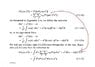# Derivation of Sudarshan-Glauber P function

• Muthumanimaran
In summary, the conversation discusses a coursework on Quantum Optics and the derivation of the P-function from the book Quantum Optics by Marlan O.Scully and M. Suhail Zubairy. The relevant equations and a part of the solution are provided in the conversation. The conversation also includes a question about expanding a function, but the solution is straightforward and easily derived from the given equations.

## Homework Statement

It is not a problem, But I am doing a coursework on Quantum Optics on my own. The following derivation is for P-function in Quantum optics from the book Quantum Optics by Marlan O.Scully and M. Suhail Zubairy. I attached the Image of the derivation with this Question.

## Homework Equations

Relevant Equations are given in the Image

## The Attempt at a Solution

I expanded the trace in the (3.1.11) in |α> basis. But I am unable to get (3.1.13).

#### Attachments

•P-function.jpg
38.3 KB · Views: 492
Muthumanimaran said:

## Homework Statement

It is not a problem, But I am doing a coursework on Quantum Optics on my own. The following derivation is for P-function in Quantum optics from the book Quantum Optics by Marlan O.Scully and M. Suhail Zubairy. I attached the Image of the derivation with this Question.

## Homework Equations

Relevant Equations are given in the Image

## The Attempt at a Solution

I expanded the trace in the (3.1.11) in |α> basis. But I am unable to get (3.1.13).
They are just saying that (by definition),

$$\int d^2 \alpha ~~\delta(a- \alpha) \delta(a^\dagger - \alpha^*) f(\alpha) f(\alpha^*) = f(a) f(a^\dagger)$$

Yeah I absolutely understand that, but I don't know how to expand f(a)f(a†). Can you help me with this?

Muthumanimaran said:
Yeah I absolutely understand that, but I don't know how to expand f(a)f(a†). Can you help me with this?
Ah, but then 3.1.13 follows directly from 3.1.11 with the choice ## f(a) = a^m## and ## f(a^\dagger) = (a^\dagger)^n##. That's all there is to it. I am not sure what the question is, then.

Oh yes, I confused myself a bit. Now I got. Its pretty straightforward. Thank you.